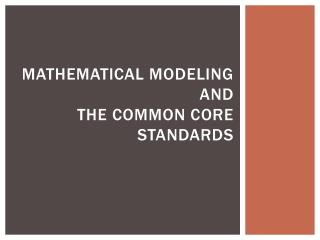DownloadDownload PresentationMathematical Modeling and the Common Core Standards

# Mathematical Modeling and the Common Core Standards

Download Presentation## Mathematical Modeling and the Common Core Standards

- - - - - - - - - - - - - - - - - - - - - - - - - - - E N D - - - - - - - - - - - - - - - - - - - - - - - - - - -
##### Presentation Transcript

1. Mathematical Modelingandthe Common Core Standards

2. Modeling is one of the eight standards for mathematical practice: 4. Model with mathematics. Mathematically proficient students can apply the mathematics they know to solve problems arising in everyday life, society, and the workplace. ….. They routinely interpret their mathematical results in the context of the situation and reflect on whether the results make sense, possibly improving the model if it has not served its purpose.

3. Modeling is one of the six conceptual categories among the high school standards The high school conceptual categories are • Number and Quantity • Algebra • Functions • Modeling • Geometry • Statistics and Probability

4. PARCC Assessment Framework will test modeling in grades 9, 10, 11 • Grade 9: • functions, function notation, interpret functions, analyze functions • construct linear, quadratic, and exponential models • summarize, represent, and interpret data on two quantitative variables • Interpret linear (regression) models

5. PARCC Assessment Framework will test modeling in grades 9, 10, 11 • Grade 10 • Apply geometric concepts in modeling situations

6. PARCC Assessment Framework will test modeling in grades 9, 10, 11 • Grade 11 • Build a function that models a relationship between two quantities • Interpret expressions for functions in terms of the situation they model